#### You may also like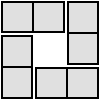### 4 Dom

Use these four dominoes to make a square that has the same number of dots on each side.### Just Rolling Round

P is a point on the circumference of a circle radius r which rolls, without slipping, inside a circle of radius 2r. What is the locus of P?### Areas and Ratios

Do you have enough information to work out the area of the shaded quadrilateral?

# Mapping the Territory

##### Age 14 to 18Challenge Level

Thank you to Navjot from Sherborne Qatar and Timothy from Tanglin Trust School in Singapore for the solutions that they submitted. The solution below draws on their observations and explanations.

Multiplying two complex numbers to get a real number
For a fixed $z_1$, you can move $z_2$ about until $z_3$ is on the real axis. For example: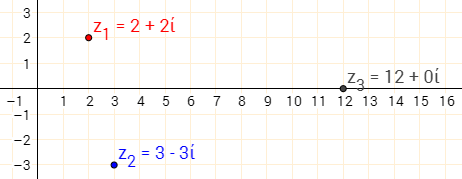$(2+2i)(3-3i)=6+6i-6i-6i^2$
$=6+6=12$

Notice now that moving $z_2$ towards $0+0i$ moves $z_3$ along the real axis.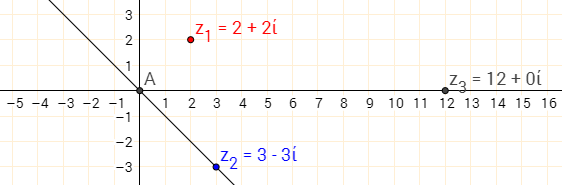$(2+2i)(3-3i)=12$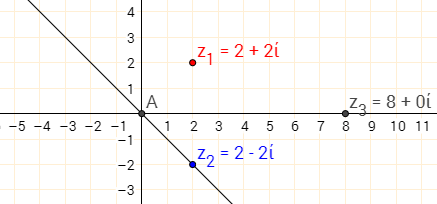$(2+2i)(2-2i)=4+4i-4i-4i^2$
$=4+4=8$

Above, $z_2$ is the reflection of $z_1$ in the real axis!

Below are more examples shown for a different $z_1$, where $z_2$ is the reflection of $z_1$ in the real axis. Note that moving $z_2$ closer to the origin along the same line through the origin also gives a real number. This is because real multiples of $z_2$ lie on this line - this is just an enlargement of $z_2$ with a real scale factor.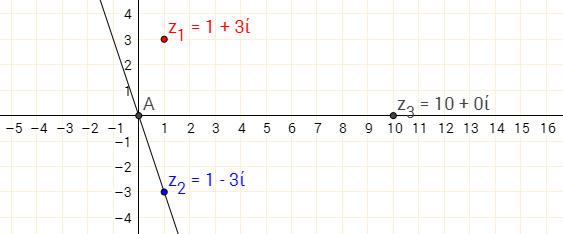$(1+3i)(1-3i)=1+3i-3i-9i^2=10$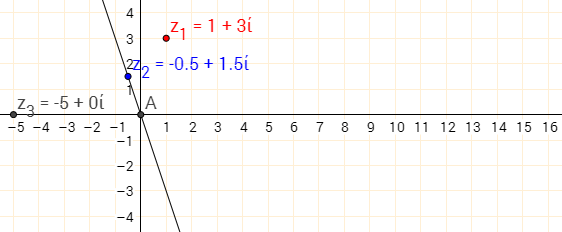$(1+3i)(-0.5+1.5i)=-0.5-1.5i+1.5i+4.5i^2=-5$

Also, $(1+3i)(-0.5+1.5i)=(1+3i)(-0.5(1-3i))$
$=-0.5(1+3i)(1-3i)$
$=-0.5\times 10$
$=-5$

Multiplying two complex numbers to get an imaginary number
This time, swapping the coefficients of the real and imaginary parts of $z_1$ works. This is the same as reflecting $z_1$ in the line "$y=x$" (shown dotted in green):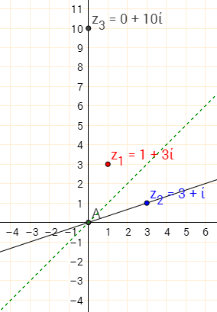$(1+3i)(3+i)=3+9i+i+3i^2$
$=3-3+9i+i =10i$

And again, real multiples of this complex number also 'work':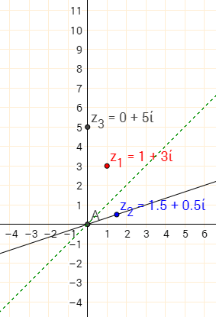$(1+3i)(1.5+0.5i)=1.5+4.5i+0.5i+1.5i^2$
$=1.5-1.5+4.5i+0.5i =5i$

Also, $(1+3i)(1.5+0.5i)=(1+3i)(0.5(3+i))$
$=0.5(1+3i)(3+i)$
$=0.5\times10=5$

Why does this happen?
Geometrically, recall from Into the Wilderness that multiplying by a complex number $z_2$ has the effect of a rotation on $z_1$.
In this case, when a line segment is drawn connecting $0+0i$ to $z_1$, we can see that in this case, the angle from the positive real axis to $z_1$ is $71.57^\circ$ anticlockwise. So it needs to be rotated by $71.57^\circ$ clockwise to land on the real axis: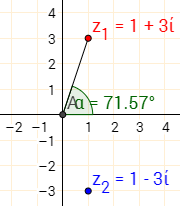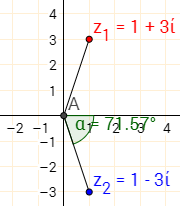Since $z_2$ is the reflection of $z_1$ in the real axis, it also makes an angle of $71.57^\circ$ with the real axis, but measured clockwise.

So multiplying $z_1$ by its reflection in the real axis is the same as rotating $z_1$ by the angle which brings it to the real axis, resulting in a real number.

Algebraically, if $z_1=a+bi$ then the reflection of $z_1$ in the real axis is $a-bi$. \begin{align}(a+bi)(a-bi)&=a^2+abi-abi-bi^2\\ &=a^2+b^2\end{align}which is real.

Mutliplying $a+bi$ by a real multiple of $a-bi$ is the same as doing, for some real $k$, $$(a+bi)(ak-bki)=k\left((a+bi)(a-bi)\right)=k(a^2+b^2)$$

And to check that there are no other possibilities, suppose $z_1=a+bi$, $z_2=c+di$, and $z_1z_2$ is real. Then: \begin{align}(a+bi)(c+di)&=r+0i\\ \Rightarrow ac+bci+adi+dbi^2&=r+0i\\ \Rightarrow (bc+ad)i&=0i\\ \Rightarrow bc&=-ad\\ \Rightarrow \frac ca&=-\frac db\end{align} So if $\dfrac ca=-\dfrac db=k$ for some real $k$ (since $a,b,c,d$ are all real), then $c=ka$ and $d=-kb$. So $c+di=k(a-bi)$.

To get an imaginary number
Geometrically, there are two ways of looking at it. Since multiplication corresponds to a rotation, we could consider the angle that $z_1$ needs to be rotated by to reach the imaginary axis: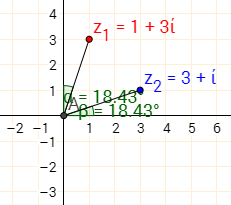Here, the horizontal distance from $0+0i$ to $z_1$ is the same as the vertical distance from $0+0i$ to $z_2$, and vice versa. This is why the angle between $z_1$'s line and the imaginary axis is the same as the angle between the real axis and $z_2$'s line.

Alternatively, we could use the result for real products: find $z_2$ such that the product is real, and then multiply by $i$. In this case, to get a real number, $z_1=1+3i$ could be multiplied by $z_2=1-3i$ to get a real number, so to get an imaginary number, is should be multiplied by $iz_2=i(1-3i)=3+i$, shows as $z_4$ below. $iz_2$ is the image of $z_2$ under a rotation $90^\circ$ anticlockwise about the origin: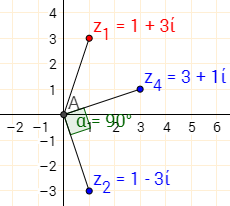Algebraically, we can use this second idea. Since we know that multiplying $a+bi$ by real multiples of $a-bi$ gives us real numbers, we know that multiplying $a+bi$ by imaginary multiples of $a-bi$ gives us imaginary numbers!

That is the same as multiplying by real multiples of $i(a-bi)=ai-bi^2=b+ai$ - swapping the coefficients $a$ and $b$.

So multiplying $a+bi$ by $k(b+ai)$ for any real $k$ will give an imaginary number.

We could also use algebra similar to the algebra above to derive this result.

To get another complex number $z_3=x+yi$
Geometrically
Thinking again geometrically, consider the yellow angle from $z_1$ to $z_3$ on the diagram below. This should be the angle from the positive real axis to $z_2$, as we found in Into the Wilderness: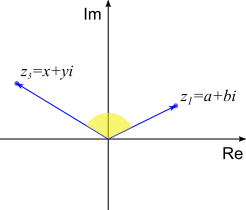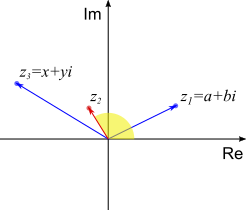And the distance from the origin to $z_2$ should be such that the products of the distances from the origin to $z_1$ and to $z_2$ is equal to the distance from the origin to $z_3$, as seen in Into the Wilderness.

Algebraically
• You could say that, if $z_1z_2=z_3$, then $z_2=\dfrac{z_3}{z_1}=\dfrac{x+yi}{a+bi}$, and then find something to multiply the numerator and denominator of this fraction by so that the denominator is a real number
(For this to work, $z_1$ has to be non-zero. What happens to $z_3$ is $z_1=0+0i$?)
• You could let $z_2=c+di$, so $(a+bi)(c+di)=x+yi$, expand the brackets and compare coefficients.
Amrit from Hymers College in this UK used this method, and then represented the complex numbers as vectors. This allowed Amrit to find the position of the complex number $c+di$ and to construct it using a ruler and a compass. Well done Amrit!
• You know that to get $x$, you need to multiply $a+bi$ by $k(a-bi)$ for some real $k$, and to get $yi$, you need to multiply $a+bi$ by $l(b+ai)$ for some real $l$. So you could find $k$ and $l$ and then add $k(a-bi)$ and $l(b+ai)$ to get $z_2$
• You could use the yellow angle and red length in the diagram above to find the coordinates of $z_3$